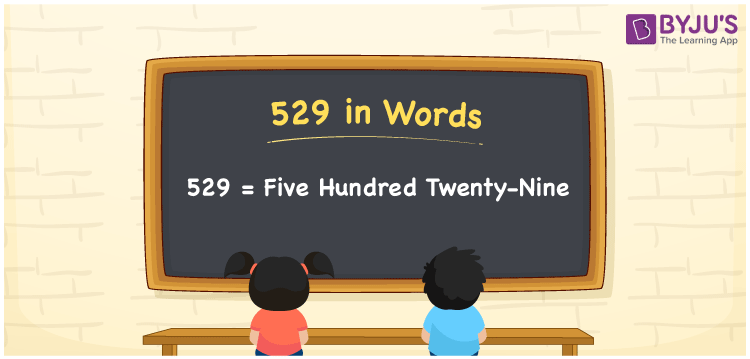# 529 in Words

529 in words is expressed as Five Hundred Twenty-Nine. For example, if the cost of 500 gm dry fruits is Rs. 529, then you can say, “The cost of a 500 gm dry fruits is Rupees Five Hundred Twenty-Nine”. 529 is a cardinal number since it represents some amount or value. In this article, we will learn how to spell 529 in English as well as how to write its numerical name with the help of a place value chart.

 529 in Words Five Hundred Twenty-Nine Five Hundred Twenty-Nine in numerical form 529

## 529 in English Words

English is the vital language used in education. Hence, generally, we write numbers in words with the help of the English alphabet. Therefore, the number 529 in English is written as Five Hundred Twenty-Nine.## How to Write 529 in Words?

To express the place value chart for 529, we need to make a place value table of three columns as it is a three-digit number. The following table shows the place value chart for 529.

 Hundreds Tens Ones 5 2 9

Hence, we can write the expanded form as:

5 x Hundred + 2 x Ten + 9 x One

= 5 x 100 + 2 x 10 + 9 x 1

= 500 + 20 + 9

= 529

= Five Hundred Twenty-Nine

Therefore, 529 in words is written as Five Hundred Twenty-Nine

Interesting way of writing 529 in words

5 = Five

52 = Fifty-Two

529 = Five Hundred Twenty-Nine

Thus, the word form of the number 529 is Five Hundred Twenty-Nine

529 is a natural number that is one less than 530 and one greater than 528

• 529 in words – Five Hundred Twenty-Nine
• Is 529 an odd number? – Yes
• Is 529 an even number? – No
• Is 529 a perfect square number? – Yes
• Is 529 a perfect cube number? – No
• Is 529 a prime number? – No
• Is 529 a composite number? – Yes

## Frequently Asked Questions on 529 in Words

Q1

### How do you write 529 in words?

529 in words is written as Five Hundred Twenty-Nine.
Q2

### Simplify 400 + 129, and express in words.

Simplifying 400 + 129, we get 529. Hence, the number 529 in words is Five Hundred Twenty-Nine.
Q3

### Is 529 an odd number?

Yes, the number 529 is an odd number.